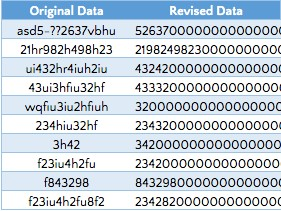NON-NUMERIC CHARACTERS in Excel
Tuesday, Jul 14# How to STRIP NON-NUMERIC CHARACTERS in Excel

There is a string of formulas that can be written to strip out only the non-numerical values from a cell and only return the numerical values. This is useful in situations where you need to calculate the sum of data contained in a cell that has non-numeric values or if you need only numeric portions of a text string, e.g. for serial numbers or other purposes.

Formula explanation

Syntax:
=TEXTJOIN(“”,TRUE,IFERROR(MID(A1,ROW(INDIRECT(“1:100″)),1)+0,””))

In the above formula, every item except A1 will be the same for every situation. Instead of typing A1, you would select the cell containing the value you would like to strip.

This formula uses the MID formula which works from the inside out to remove non-numeric characters.

The ROW and INDIRECT functions act to reference an array of the numbers between 1 and 100.

Example:

We have the below raw data in the “Original Data” column and would like to make a column of only the numeric values beside it in the “Revised Data” column.Overall, this is a great formula to use to strip out the numerical values ONLY!

I hope that helps. Please leave a comment below with any questions or suggestions. For more in-depth Excel training, checkout our Ultimate Excel Training Course here. Thank you!

Article RatingInline Feedbacks